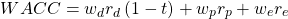Concept 61: Weighted Average Cost of Capital (WACC) | IFT World
101 Concepts for the Level I Exam

# Concept 61: Weighted Average Cost of Capital (WACC)• It is the overall cost of the sources of capital.
• Represents the required return or opportunity costs for the firm as a whole.
• It is the appropriate discount rate for cash flows of projects having similar risk profile as that of the firm.
• Has weights that are derived from target capital structure and market values of each source of capital.
• Is also called the marginal cost of capital (MCC)A firm has the following capital structure: 20% debt, 10% preferred stock, and 70% equity. The before-tax cost of debt is 6%, cost of preferred stock is 8%, and cost of equity is 12%. The firm’s marginal tax rate is 30 percent. Calculate its WACC.

Solution:

The WACC can be calculated using the formula.

WACC = (0.2) (0.06) (1 – 0.3) + (0.1) (0.08) + (0.7) (0.12) = 10.04%

** IFT Website Un-available on Monday 2-October-2023, 0700-1200 GMT **
This is default text for notification bar